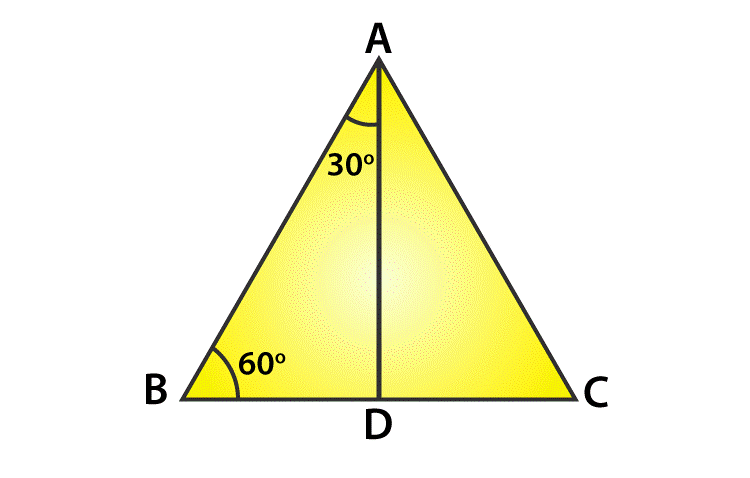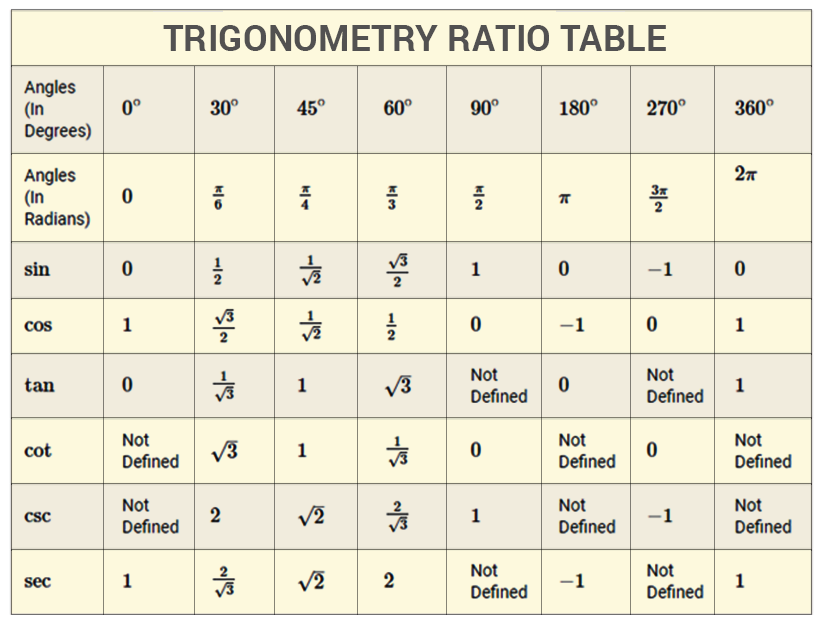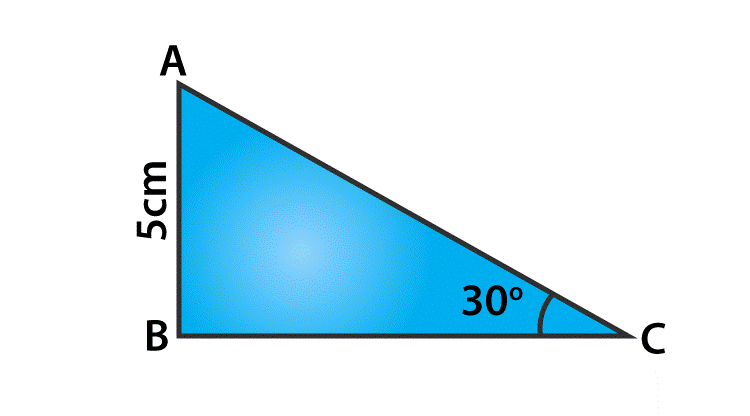# Sin 30 degrees

The trigonometric functions also called as an angle function that relates the angles of a triangle to the length of its sides. Trigonometric functions are most important in the study of periodic phenomena like sound and light waves, average temperature variations and the position and velocity of harmonic oscillators and many other applications. The most familiar three trigonometric ratios are
sine function, cosine function and tangent function. For angles less than a right angle, trigonometric functions are commonly defined as the ratio of two sides of a right triangle containing the angle and their values can be found in the length of various line segments around a unit circle. The angles are calculated with respect to sin, cos and tan functions. Usually, the degrees are considered as 0°, 30°, 45°, 60°, 90°, 180°, 270° and 360°. Here, we will discuss the value for sin 30 degrees and how to derive the sin 30 value using other degrees or radians.

## Sine 30 Degrees Value

To define the sine function of an acute angle, start with the right-angled triangle ABC with the angle of interest and the sides of a triangle. The three sides of the triangle are given as follows:

• The opposite side is the side opposite to the angle of interest.
• The hypotenuse side is the side opposite the right angle and it is always the longest side of a right triangle
• The adjacent side is the remaining side and it forms a side of both the angle of interest and the right angle

The sine function of an angle is equal to the length of the opposite side divided by the length of the hypotenuse side and the formula is given by

$\sin \theta =\frac{opposite side}{hypotenuse side}$

The sine law states that the sides of a triangle are proportional to the sine of the opposite angles.

$\frac{a}{\sin A}=\frac{b}{\sin B}=\frac{c}{\sin C}$

The sine rule is used in the following cases :

Case 1: Given two angles and one side (AAS and ASA)

Case 2: Given two sides and non included angle (SSA)

## Derivation to Find the Sin 30 value

Let us now calculate the sin 30 value. Consider an equilateral triangle ABC. Since each angle in an equilateral triangle is 60°, therefore $\angle A=\angle B=\angle C=60^{\circ}$Draw the perpendicular line AD from A to the side BC (From figure)

Now $\Delta ABD\cong \Delta ACD$

Therefore BD=DC and also

$\angle BAD=\angle CAD$

Now observe that the triangle ABD is a right triangle, right-angled at D with $\angle BAD=30^{\circ}$ and $\angle ABD=60^{\circ}$.

As you know, for finding the trigonometric ratios, we need to know the lengths of the sides of the triangle. So, let us suppose that AB=2a

$BD=\frac{1}{2}BC=a$

To find the sin 30-degree value, let’s use sin 30-degree formula and it is written as

Sin 30° = opposite side/hypotenuse side

We know that, Sin 30° = BD/AB = a/2a = 1 / 2

Therefore, Sin 30 degree equals to the fractional value of 1/ 2.

Sin 30° = 1 / 2

Therefore, sin 30 value is 1/2

In the same way, we can derive other values of sin degrees like 0°, 30°, 45°, 60°, 90°,180°, 270° and 360°. Below is the trigonometry table, which defines all the values of sine along with other trigonometric ratios.### Sample Example

Question:

In triangle ABC, right-angled at B, AB = 5 cm and angle ACB = 30°. Determine the length of the side AC.Solution:

To find the length of the side AC, we consider the sine function, and the formula is given by

Sin 30°= Opposite Side / Hypotenuse side

Sin 30°= AB / AC

Substitute the sin 30 value and AB value,

$\frac{1}{2}=\frac{5}{AC}$

Therefore, the length of the hypotenuse side, AC = 10 cm.

Keep visiting BYJU’S for more information on trigonometric ratios and its related articles, and also watch the videos to clarify the doubts.

 Related Links Sin 0 Degree Trigonometry Formulas Inverse Cosine Law of Sines Formula